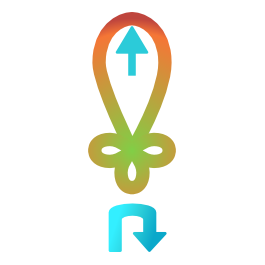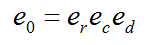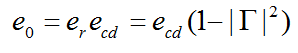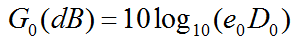#### Realised gain calculatorThe realised gain of an antenna is calculated by considering the total efficiency of the antenna, along with its directivity.

The total efficiency of the antenna considers the losses due to reflections at the input terminals as well as losses within the structure of the antenna.

Total efficiency e0 may be written aswhere er is the reflection (mismatch) efficiency, ed the dielectric efficiency and ec the conduction efficiency. The dielectric and conduction efficiencies are usually grouped, since they are measured as one entity, and known as ecd. Total efficiency may therefore be written as:The realised gain is calculated using the total efficiency and the directivity as follows:Realised gain and directivity are specified in dBi, the voltage reflection coefficient in dB, and the efficiency as a value between 0 and 1.